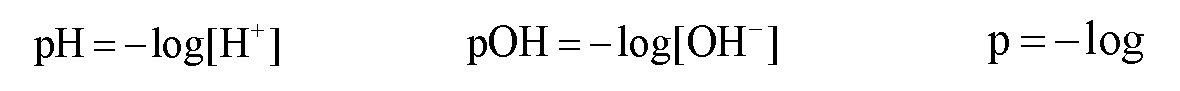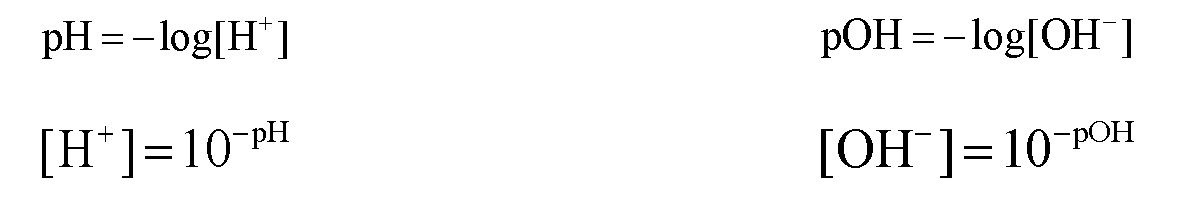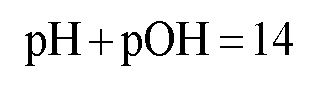Clutch Prep is now a part of Pearson
Ch.10 Acids and BasesWorksheetSee all chapters

# The pH Scale

See all sections
Sections
Acid-Base Introduction
Arrhenius Acid and Base
Bronsted Lowry Acid and Base
Acid and Base Strength
Ka and Kb
The pH Scale
Auto-Ionization
pH of Strong Acids & Bases
Acid-Base Equivalents
Acid-Base Reactions
Gas Evolution Equations (Simplified)
Ionic Salts (Simplified)
Buffers
Henderson-Hasselbalch Equation
Strong Acid Strong Base Titrations (Simplified)

The pH and pOH of a compound helps to determine its acidity and basicity.

###### The pH Scale

Concept #1: Understanding pH vs. pOH.

Transcript

Hey guys, in this new video we’re going to take a closer look at pH and pOH. We’re going to say that when it comes to the concentrations of H+ and OH-, they usually come as very small numbers. What we need to realize here is that the pH scale was invented to basically turn these varying small numbers into more manageable ones. We’re going to say under normal conditions, the pH scale operates within the range of 0 to 14. But what we need to realize here is that the pH scale could be less than 0 or could be greater than 14. All we would need to do is increase the concentration of our acid or base. Remember, 0 to 14 are not the only numbers that your pH or pOH could be. They could be greater or less than that. It all again depends on upping the concentration of your compound. If you make your concentration very high like over 1 molar, then it will give you a very high or very low pH.
We’re going to say that by taking the negative log of H+ and OH-, we can find pH and pOH. pH just means negative log of H+ concentration or negative log of H3O+. Because remember we said this before H+, H3O+ same exact thing. pOH is the negative log of OH-, so that means that p represents negative log.

pH stands for the negative logarithmic function of your hydronium concentration, whereas pOH stands for the negative logarithmic function of hydroxide concentration.Concept #2: The connection to Hydronium ion and Hydroxide ion concentrations.

Transcript

What we should realize here is that by recognizing the relationship between H+ and OH-, we can find a new way to express them in terms of pH and pOH. We come back here. We say that pH equals negative log of H+. We could divide both sides by -1 to get rid of this negative, so –pH equals a log of H+. We want to just isolate the H+ so we’re going to divide out the log. When you divide anything by log, it becomes 10 to that whatever the variable is.
We just found a new relationship. We can say that H+, the concentration of H+ ions is equal to 10 to the negative pH. We could do the same thing with pOH. Divide both sides by -1 where -pOH equals log of OH-. Divide both sides by log, so OH- equals 10 to the negative pOH. So, just remember the relationship that we have here with these first two equations and how we have the relationship with their concentrations. Because sometimes you’ll be asked to find H+ concentration if they give you pH. Sometimes you may be asked to find OH- concentration if they give you pOH. You have to know the relationship both frontwards and backwards.

If we know the pH or pOH concentration then we can determine the concentration of hydronium ions or hydroxide ions.Concept #3: The pH Scale

Transcript

We’re going to say a species with a pH greater than 7 is classified as basic. If you’re basic, you’re going to say that your H+ concentration is less than your OH- concentration. We can also say that the stronger the base, then the higher the pH. The higher the pH, the greater your hydroxide concentration. We can understand this relationship as strongest base would have the highest pH and therefore have the highest OH- concentration. But remember, we already went over this several videos ago. How do we determine if something is weak or strong? You still need to know those in order to do the following questions because again, I’ve said it so many times before, why do we need to know the strength of things? Because eventually we’re going to have to use ICE charts to find pH if we’re given a weak acid or a weak base. That’s why it’s important to know what our species is – is it weak or is it strong.
We’re going to say a species with a pH less than 7 is termed acidic. If you’re acidic, your H+ concentration is greater than your OH- concentration. Here we can say the stronger the acid then the lower the pH. Strongest acid has the lowest pH which means you have the highest H+.
Then finally we’re going to say a species with a pH equal to 7 is neutral. Remember, this is only true at 25°Celsius because remember at 25°Celsius KW= 1.0 times ten to the negative 14. If they were to change the temperature, that would change our KW. The neutral pH would become a new number. It doesn’t always equal 7. We’re going to say here if you’re neutral and your H+ concentration is equal to your OH- concentration.

If the pH is less than 7 then the solution is acidic, if the pH is equal to 7 then the solution is neutral and if the pH is greater than 7 then the solution is basic.

Concept #4: The pH and pOH connection.

Transcript

And we're going to say here, we talked about pH and pOH and just realize their relationship is connected together by this equation, we're going to say pH plus pOH equals 14, this arises by the fact that we have Kw equals H+ times OH minus and if you take the negative log of H+ it gives you pH, if you take the negative log of OH- it gives you pOH and Kw is 1.0 times 10 to the negative 14 and if you took the negative log of Kw it would give you 14. So, that's where this equation comes from, it comes from taking the negative log of all of this, and remember, if you have two things multiplying like H+ and OH- are multiplying and you take the negative log of that whole thing then it becomes the addition between them, okay? So, these guys are related, these two equations that I circled are related because they're connected together by negative log. So, just remember that relationship and you'll be able to remember this formula and remember pH scale 0 to 14, you can be below 0 or higher than 14 depending on the concentration of the acid or base you're using.

Under normal conditions when the concentration is less than 1.0 M the pH scale is between 0 to 14. pH and pOH are connected by the following equation:Example #1: What is the hydroxide ion and hydronium ion concentration of an aqueous solution that has a pH equal to 6.12?

Transcript

Hey guys, in this new video, we’re going to put to practice some of the concepts we learned about pH and pOH. Let’s take a look at the first example. Here it says what is the hydroxide ion, so what’s the OH-, and the hydronium ion concentration, that’s H+, of an aqueous solution that has a pH equal to 6.12? We have pH. We need to find OH- and H+. Remember we’re able to derive a formula that connects us between H+ and pH. Remember that H+ equals 10 to the negative pH. All we have to do now is take that number and plug it in. When we do that, we get 7.59 times ten to the negative 7.
We could find OH now and we can find OH in two different ways. The first way we could find OH- is by simply using this equation: KW equals H+ times OH-. We know what KW is. We assume that the temperature is 25 degrees Celsius since they don’t tell us what it is. This would be 1.0 times 10 to the negative 14. We just found out what H+ is. It’s 7.59 times 10 to the negative seven. Now we have to find OH-. OH- is our only missing variable so just isolate it. Here, OH- would equal 1.32 times 10 to the negative 8. That would be one way we could find OH-. The second way we could find it is if we know pOH, we can make a connection between pOH and OH-. First, we’d say that pH plus pOH equals 14. We know pH is 6.12, so let’s just isolate our pOH. Subtract 6.12 from both sides so pOH equals 7.88. Remember, what’s the connection between pOH and OH-? You can just simply say that OH- equals 10 to the negative pOH. If you did 10 to the negative 7.88, you get the same exact answer for OH-.
Two different methods to find the same exact missing variable. It’s basically your choice on deciding which way you want to go. Personally, I like just find pOH and just do 10 to the negative pOH because remember, if you don’t put these two in brackets, your calculator may give you the incorrect answer. It’s probably always safer just to say OH- equals 10 to the negative pOH.

Practice: Which of the following statements about aqueous solutions is/are true?

Example #2: A solution is prepared by dissolving 0.235 mol Sr(OH)2 in water to produce a solution with a volume of 750 mL.

a) What is the [OH-]?

b) What is the [H+]?

Practice: What is the Kw of pure water at 20.0°C, if the pH is 7.083?